• 全文共4206字，预计学习时长8分钟觉得物理太难了？本文将模拟N维空间中的某些向量场(例如电磁场)，以便你更清晰地理解这些概念。理论基础向量任何物理场景的基本元素都是向量。我们需要什么？需要向量的算术运算、...
全文共4206字，预计学习时长8分钟觉得物理太难了？本文将模拟N维空间中的某些向量场(例如电磁场)，以便你更清晰地理解这些概念。理论基础向量任何物理场景的基本元素都是向量。我们需要什么？需要向量的算术运算、距离、模块以及一些技术层面的东西。从列表中得到向量。下面是它初始化的样子：class Vector(list):    def __init__(self, *el):        for e in el:            self.append(e)现在，创造一个向量：v = Vector(1, 2, 3)再进行加法算术运算：class Vector(list):...    def __add__(self, other):        if type(other) is Vector:            assert len(self) == len(other), "Error 0"            r = Vector()            for i in range(len(self)):                r.append(self[i] + other[i])            return r        else:            other = Vector.emptyvec(lens=len(self), n=other)            return self + other结果：v1 = Vector(1, 2, 3)v2 = Vector(2, 57, 23.2)v1 + v2>>> [3, 59, 26.2]同样需定义所有的算术运算(向量的完整代码放在最后)。现在需要一个距离函数。创建dist (v1, v2)并不难—但也并不美观，因此重新定义 %运算符。class Vector(list):...    def __mod__(self, other):        return sum((self - other) ** 2) ** 0.5结果：v1 = Vector(1, 2, 3)v2 = Vector(2, 57, 23.2)v1 % v2>>> 58.60068258988115还需要一些方法来快速生成向量并且完美地输出。这没有什么棘手的问题，因此以上是向量类的整个代码。粒子理论上而言，这里的一切都很简单—点具有坐标、速度及加速度。另外，它有大量的自定义参数组(例如，对于电磁场，可以设置电荷)。下面进行初始化：class Point:    def __init__(self, coords, mass=1.0, q=1.0 speed=None, **properties):        self.coords = coords        if speed is None:            self.speed = Vector(*[0 for i in range(len(coords))])        else:            self.speed = speed        self.acc = Vector(*[0 for i in range(len(coords))])        self.mass = mass        self.__params__ = ["coords", "speed", "acc", "q"] + list(properties.keys())        self.q = q        for prop in properties:            setattr(self, prop, properties[prop])以下方法可以将点移动、固定及加速：class Point:...    def move(self, dt):        self.coords = self.coords + self.speed * dt    def accelerate(self, dt):        self.speed = self.speed + self.acc * dt    def accinc(self, force):  # Considering exerted force the point obtains acceleration        self.acc = self.acc + force / self.mass    def clean_acc(self):        self.acc = self.acc *很好，这一部分已经完成。相互作用场相互作用场可称为物体，它包含来自太空的所有粒子，并且作用于这些粒子。我们将考虑宇宙的一种特殊情况，因此将进行自定义交互(当然，这易于扩展)。表明构造函数并添加点。class InteractionField:    def __init__(self, F):  # F - is a custom force, F(p1, p2, r), p1, p2 - points, r - distance inbetween        self.points = []        self.F = F    def append(self, *args, **kwargs):        self.points.append(Point(*args, **kwargs))现在，有趣的是声明一个函数，该函数在那一点返回“tension”。尽管该概念原指电磁场交互，但是在我们的情况中，它指某种抽象向量，可依据它来移动点。这样将拥有q点的属性，在特殊情况下，我们将拥有点的电荷(通常是我们想要的任何东西，甚至向量)。所以，C点的张力是多少？大概是这样：C点的张力C点的电场强度等于作用于某个单位点上所有物质点的力之和。class InteractionField:...    def intensity(self, coord):        proj = Vector(*[0 for i in range(coord.dim())])        single_point = Point(Vector(), mass=1.0, q=1.0)  # That's our "Single point"        for p in self.points:            if coord % p.coords < 10 ** (-10):  # Check whether we compare coord with a point P where P.coords = coord                continue            d = p.coords % coord            fmod = self.F(single_point, p, d) * (-1)                proj = proj + (coord - p.coords) / d * fmod         return proj这时，你已经可以将向量场可视化，但通常最后完成它。下面进行交互。class InteractionField:...    def step(self, dt):        self.clean_acc()        for p in self.points:            p.accinc(self.intensity(p.coords) * p.q)            p.accelerate(dt)            p.move(dt)对于每个点，确定这些坐标的强度，进而确定对该粒子上的最终作用力：确定对该粒子的最终作用力质点运动和向量场可视化最后，来到了最有趣的部分。开始吧！模拟电磁场中的粒子运动u = InteractionField(lambda p1, p2, r: 300000 * -p1.q * p2.q / (r ** 2 + 0.1))for i in range(3):    u.append(Vector.randvec(2) * 10, q=random.random() - 0.5)实际上，系数K应该等于数十亿(9 * 10 ^ (- 9))，但由于在时间t -> 0之前会失超，所以将它们都设为正数会更容易。因此，本物理学中，K=300,000.接下来，沿着每个轴增加10个点(二维空间)，即在坐标轴上从0到10，也给每个点设置-0.25至0.25的电荷。然后，运行一个循环，并依据其坐标(轨迹)绘制点：X, Y = [], []for i in range(130):    u.step(0.0006)    xd, yd = zip(*u.gather_coords())    X.extend(xd)    Y.extend(yd)plt.figure(figsize=[8, 8])plt.scatter(X, Y)plt.scatter(*zip(*u.gather_coords()), color="orange")plt.show(其结果应该如下：确定对该粒子的最终作用力事实上，其绘图是完全随机的，因为目前(2019年)每个点的轨迹是不可预测的。向量场可视化需要通过一些步骤来确定坐标，并且在每个坐标上绘制正确的向量。fig = plt.figure(figsize=[5, 5])res = []STEP = 0.3for x in np.arange(0, 10, STEP):    for y in np.arange(0, 10, STEP):        inten = u.intensity(Vector(x, y))        F = inten.mod()        inten /= inten.mod() * 4  # длина нашей палочки фиксирована        res.append(([x - inten / 2, x + inten / 2], [y - inten / 2, y + inten / 2], F))for r in res:    plt.plot(r, r, color=(sigm(r), 0.1, 0.8 * (1 - sigm(r)))) # Цвет по хитрой формуле чтобы добиться градиентаplt.show()应该已经获得类似这样的：第一次向量场可视化你可以加长向量本身，用* 1.5替换* 4：向量长度增加后的向量可视化程度变更维度创建具有200个点的五维空间和一个交互，该交互依赖于第四度而不是距离的平方。u = InteractionField(lambda p1, p2, r: 300000 * -p1.q * p2.q / (r ** 4 + 0.1))for i in range(200):    u.append(Vector.randvec(5) * 10, q=random.random() - 0.5)五维已定义所有的坐标、速度等。模拟如下：velmod = 0velocities = []for i in range(100):    u.step(0.0005)    velmod = sum([p.speed.mod() for p in u.points])   # Adding sum of modules of all the velocities    velocities.append(velmod)plt.plot(velocities)plt.show()给定时间的速度总和这是在任意给定时间中的速度总和。正如你所看到的，随着时间的推移，它们在缓慢加速。以上是关于简单模拟基础物理的简短操作说明。留言 点赞 关注我们一起分享AI学习与发展的干货编译组：王小燕、伍颖欣相关链接：如需转载，请后台留言，遵守转载规范
展开全文• 觉得物理太难了？本文将模拟N维空间中的某些向量场（例如电磁场），以便你更清晰地理解这些概念。 理论基础 向量 任何物理场景的基本元素都是向量。我们需要什么？需要向量的算术运算、距离、模块以及一些...
全文共4206字，预计学习时长8分钟觉得物理太难了？本文将模拟N维空间中的某些向量场（例如电磁场），以便你更清晰地理解这些概念。理论基础

向量

任何物理场景的基本元素都是向量。我们需要什么？需要向量的算术运算、距离、模块以及一些技术层面的东西。从列表中得到向量。下面是它初始化的样子：

class Vector(list):
def __init__(self, *el):
for e in el:
self.append(e)

现在，创造一个向量：

v = Vector(1, 2, 3)

再进行加法算术运算：

class Vector(list):
...
def __add__(self, other):
if type(other) is Vector:
assert len(self) == len(other), "Error 0"
r = Vector()
for i in range(len(self)):
r.append(self[i] + other[i])
return r
else:
other = Vector.emptyvec(lens=len(self), n=other)
return self + other

结果：

v1 = Vector(1, 2, 3)
v2 = Vector(2, 57, 23.2)
v1 + v2
>>> [3, 59, 26.2]

同样需定义所有的算术运算（向量的完整代码放在最后）。现在需要一个距离函数。创建dist (v1, v2)并不难—但也并不美观，因此重新定义 %运算符。

class Vector(list):
...
def __mod__(self, other):
return sum((self - other) ** 2) ** 0.5

结果：

v1 = Vector(1, 2, 3)
v2 = Vector(2, 57, 23.2)
v1 % v2
>>> 58.60068258988115

还需要一些方法来快速生成向量并且完美地输出。这没有什么棘手的问题，因此以上是向量类的整个代码。

粒子

理论上而言，这里的一切都很简单—点具有坐标、速度及加速度。另外，它有大量的自定义参数组（例如，对于电磁场，可以设置电荷）。

下面进行初始化：

class Point:
def __init__(self, coords, mass=1.0, q=1.0 speed=None, **properties):
self.coords = coords
if speed is None:
self.speed = Vector(*[0 for i in range(len(coords))])
else:
self.speed = speed
self.acc = Vector(*[0 for i in range(len(coords))])
self.mass = mass
self.__params__ = ["coords", "speed", "acc", "q"] + list(properties.keys())
self.q = q
for prop in properties:
setattr(self, prop, properties[prop])

以下方法可以将点移动、固定及加速：

class Point:
...
def move(self, dt):
self.coords = self.coords + self.speed * dt
def accelerate(self, dt):
self.speed = self.speed + self.acc * dt
def accinc(self, force): # Considering exerted force the point obtains acceleration
self.acc = self.acc + force / self.mass
def clean_acc(self):
self.acc = self.acc *

很好，这一部分已经完成。

相互作用场

相互作用场可称为物体，它包含来自太空的所有粒子，并且作用于这些粒子。我们将考虑宇宙的一种特殊情况，因此将进行自定义交互（当然，这易于扩展）。表明构造函数并添加点。

class InteractionField:
def __init__(self, F): # F - is a custom force, F(p1, p2, r), p1, p2 - points, r - distance inbetween
self.points = []
self.F = F
def append(self, *args, **kwargs):
self.points.append(Point(*args, **kwargs))

现在，有趣的是声明一个函数，该函数在那一点返回“tension”。尽管该概念原指电磁场交互，但是在我们的情况中，它指某种抽象向量，可依据它来移动点。这样将拥有q点的属性，在特殊情况下，我们将拥有点的电荷（通常是我们想要的任何东西，甚至向量）。所以，C点的张力是多少？大概是这样：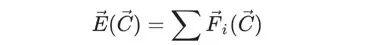C点的张力

C点的电场强度等于作用于某个单位点上所有物质点的力之和。

class InteractionField:
...
def intensity(self, coord):
proj = Vector(*[0 for i in range(coord.dim())])
single_point = Point(Vector(), mass=1.0, q=1.0) # That's our "Single point"
for p in self.points:
if coord % p.coords < 10 ** (-10): # Check whether we compare coord with a point P where P.coords = coord
continue
d = p.coords % coord
fmod = self.F(single_point, p, d) * (-1)
proj = proj + (coord - p.coords) / d * fmod
return proj

这时，你已经可以将向量场可视化，但通常最后完成它。下面进行交互。

class InteractionField:
...
def step(self, dt):
self.clean_acc()
for p in self.points:
p.accinc(self.intensity(p.coords) * p.q)
p.accelerate(dt)
p.move(dt)

对于每个点，确定这些坐标的强度，进而确定对该粒子上的最终作用力：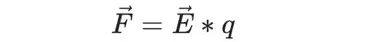确定对该粒子的最终作用力

质点运动和向量场可视化

最后，来到了最有趣的部分。开始吧！

模拟电磁场中的粒子运动

u = InteractionField(lambda p1, p2, r: 300000 * -p1.q * p2.q / (r ** 2 + 0.1))
for i in range(3):
u.append(Vector.randvec(2) * 10, q=random.random() - 0.5)

实际上，系数K应该等于数十亿(9 * 10 ^ (- 9))，但由于在时间t -> 0之前会失超，所以将它们都设为正数会更容易。因此，本物理学中，K=300,000.

接下来，沿着每个轴增加10个点（二维空间），即在坐标轴上从0到10，也给每个点设置-0.25至0.25的电荷。然后，运行一个循环，并依据其坐标（轨迹）绘制点：

X, Y = [], []
for i in range(130):
u.step(0.0006)
xd, yd = zip(*u.gather_coords())
X.extend(xd)
Y.extend(yd)
plt.figure(figsize=[8, 8])
plt.scatter(X, Y)
plt.scatter(*zip(*u.gather_coords()), color="orange")
plt.show(

其结果应该如下：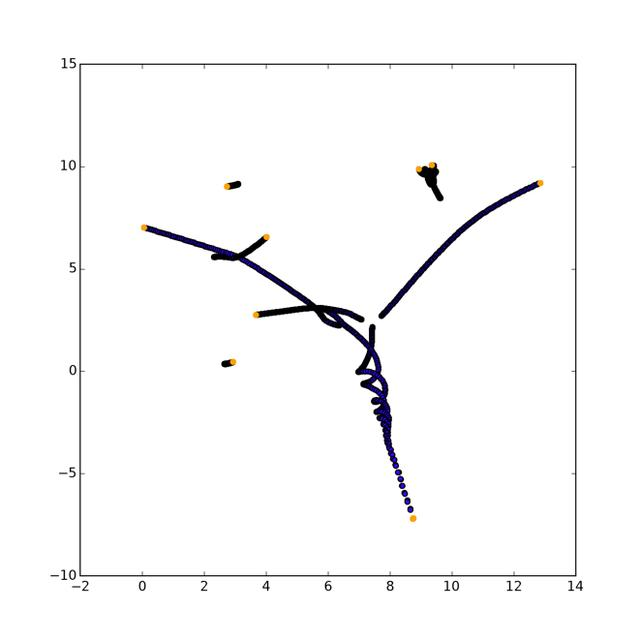确定对该粒子的最终作用力

事实上，其绘图是完全随机的，因为目前（2019年）每个点的轨迹是不可预测的。

向量场可视化

需要通过一些步骤来确定坐标，并且在每个坐标上绘制正确的向量。

fig = plt.figure(figsize=[5, 5])
res = []
STEP = 0.3
for x in np.arange(0, 10, STEP):
for y in np.arange(0, 10, STEP):
inten = u.intensity(Vector(x, y))
F = inten.mod()
inten /= inten.mod() * 4 # длина нашей палочки фиксирована
res.append(([x - inten / 2, x + inten / 2], [y - inten / 2, y + inten / 2], F))
for r in res:
plt.plot(r, r, color=(sigm(r), 0.1, 0.8 * (1 - sigm(r)))) # Цвет по хитрой формуле чтобы добиться градиента
plt.show()

应该已经获得类似这样的：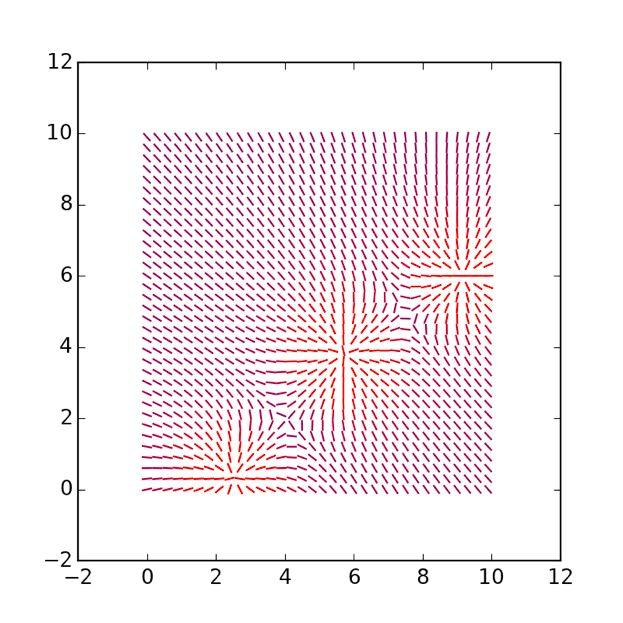第一次向量场可视化

你可以加长向量本身，用* 1.5替换* 4：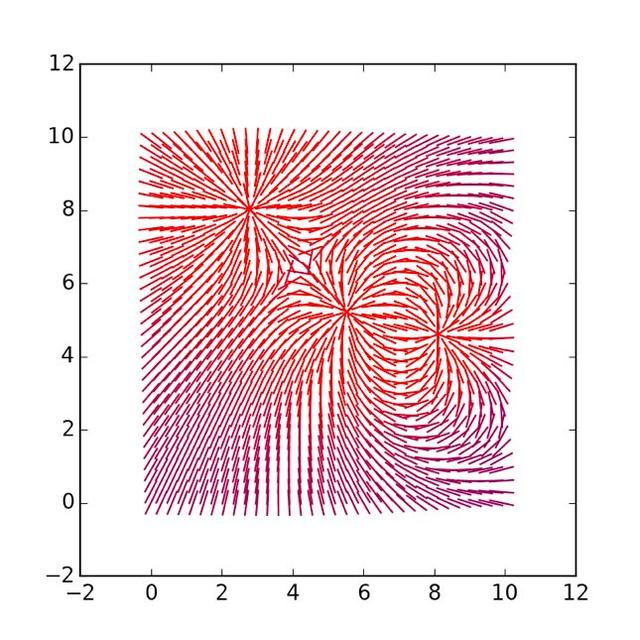向量长度增加后的向量可视化程度

变更维度

创建具有200个点的五维空间和一个交互，该交互依赖于第四度而不是距离的平方。

u = InteractionField(lambda p1, p2, r: 300000 * -p1.q * p2.q / (r ** 4 + 0.1))
for i in range(200):
u.append(Vector.randvec(5) * 10, q=random.random() - 0.5)

五维已定义所有的坐标、速度等。

模拟如下：

velmod = 0
velocities = []
for i in range(100):
u.step(0.0005)
velmod = sum([p.speed.mod() for p in u.points]) # Adding sum of modules of all the velocities
velocities.append(velmod)
plt.plot(velocities)
plt.show()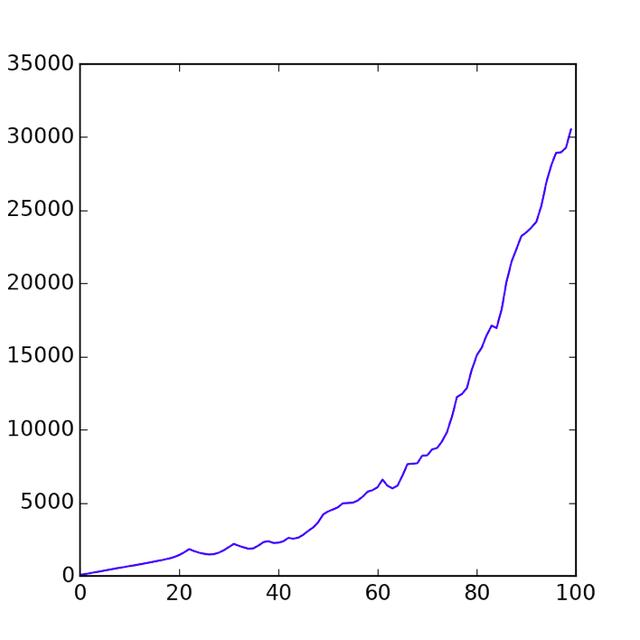给定时间的速度总和

这是在任意给定时间中的速度总和。正如你所看到的，随着时间的推移，它们在缓慢加速。

以上是关于简单模拟基础物理的简短操作说明。留言 点赞 关注

我们一起分享AI学习与发展的干货
欢迎关注全平台AI垂类自媒体 “读芯术”（添加小编微信：dxsxbb，加入读者圈，一起讨论最新鲜的人工智能科技哦～）

展开全文人工智能 Python
• 全文共4206字，预计学习时长8分钟觉得物理太难了？本文将模拟N维空间中的某些向量场(例如电磁场)，以便你更清晰地理解这些概念。理论基础向量任何物理场景的基本元素都是向量。我们需要什么？需要向量的算术运算、...
全文共4206字，预计学习时长8分钟觉得物理太难了？本文将模拟N维空间中的某些向量场(例如电磁场)，以便你更清晰地理解这些概念。理论基础向量任何物理场景的基本元素都是向量。我们需要什么？需要向量的算术运算、距离、模块以及一些技术层面的东西。从列表中得到向量。下面是它初始化的样子：class Vector(list):    def __init__(self, *el):        for e in el:            self.append(e)现在，创造一个向量：v = Vector(1, 2, 3)再进行加法算术运算：class Vector(list):...    def __add__(self, other):        if type(other) is Vector:            assert len(self) == len(other), "Error 0"            r = Vector()            for i in range(len(self)):                r.append(self[i] + other[i])            return r        else:            other = Vector.emptyvec(lens=len(self), n=other)            return self + other结果：v1 = Vector(1, 2, 3)v2 = Vector(2, 57, 23.2)v1 + v2>>> [3, 59, 26.2]同样需定义所有的算术运算(向量的完整代码放在最后)。现在需要一个距离函数。创建dist (v1, v2)并不难—但也并不美观，因此重新定义 %运算符。class Vector(list):...    def __mod__(self, other):        return sum((self - other) ** 2) ** 0.5结果：v1 = Vector(1, 2, 3)v2 = Vector(2, 57, 23.2)v1 % v2>>> 58.60068258988115还需要一些方法来快速生成向量并且完美地输出。这没有什么棘手的问题，因此以上是向量类的整个代码。粒子理论上而言，这里的一切都很简单—点具有坐标、速度及加速度。另外，它有大量的自定义参数组(例如，对于电磁场，可以设置电荷)。下面进行初始化：class Point:    def __init__(self, coords, mass=1.0, q=1.0 speed=None, **properties):        self.coords = coords        if speed is None:            self.speed = Vector(*[0 for i in range(len(coords))])        else:            self.speed = speed        self.acc = Vector(*[0 for i in range(len(coords))])        self.mass = mass        self.__params__ = ["coords", "speed", "acc", "q"] + list(properties.keys())        self.q = q        for prop in properties:            setattr(self, prop, properties[prop])以下方法可以将点移动、固定及加速：class Point:...    def move(self, dt):        self.coords = self.coords + self.speed * dt    def accelerate(self, dt):        self.speed = self.speed + self.acc * dt    def accinc(self, force):  # Considering exerted force the point obtains acceleration        self.acc = self.acc + force / self.mass    def clean_acc(self):        self.acc = self.acc *很好，这一部分已经完成。相互作用场相互作用场可称为物体，它包含来自太空的所有粒子，并且作用于这些粒子。我们将考虑宇宙的一种特殊情况，因此将进行自定义交互(当然，这易于扩展)。表明构造函数并添加点。class InteractionField:    def __init__(self, F):  # F - is a custom force, F(p1, p2, r), p1, p2 - points, r - distance inbetween        self.points = []        self.F = F    def append(self, *args, **kwargs):        self.points.append(Point(*args, **kwargs))现在，有趣的是声明一个函数，该函数在那一点返回“tension”。尽管该概念原指电磁场交互，但是在我们的情况中，它指某种抽象向量，可依据它来移动点。这样将拥有q点的属性，在特殊情况下，我们将拥有点的电荷(通常是我们想要的任何东西，甚至向量)。所以，C点的张力是多少？大概是这样：C点的张力C点的电场强度等于作用于某个单位点上所有物质点的力之和。class InteractionField:...    def intensity(self, coord):        proj = Vector(*[0 for i in range(coord.dim())])        single_point = Point(Vector(), mass=1.0, q=1.0)  # That's our "Single point"        for p in self.points:            if coord % p.coords < 10 ** (-10):  # Check whether we compare coord with a point P where P.coords = coord                continue            d = p.coords % coord            fmod = self.F(single_point, p, d) * (-1)                proj = proj + (coord - p.coords) / d * fmod         return proj这时，你已经可以将向量场可视化，但通常最后完成它。下面进行交互。class InteractionField:...    def step(self, dt):        self.clean_acc()        for p in self.points:            p.accinc(self.intensity(p.coords) * p.q)            p.accelerate(dt)            p.move(dt)对于每个点，确定这些坐标的强度，进而确定对该粒子上的最终作用力：确定对该粒子的最终作用力质点运动和向量场可视化最后，来到了最有趣的部分。开始吧！模拟电磁场中的粒子运动u = InteractionField(lambda p1, p2, r: 300000 * -p1.q * p2.q / (r ** 2 + 0.1))for i in range(3):    u.append(Vector.randvec(2) * 10, q=random.random() - 0.5)实际上，系数K应该等于数十亿(9 * 10 ^ (- 9))，但由于在时间t -> 0之前会失超，所以将它们都设为正数会更容易。因此，本物理学中，K=300,000.接下来，沿着每个轴增加10个点(二维空间)，即在坐标轴上从0到10，也给每个点设置-0.25至0.25的电荷。然后，运行一个循环，并依据其坐标(轨迹)绘制点：X, Y = [], []for i in range(130):    u.step(0.0006)    xd, yd = zip(*u.gather_coords())    X.extend(xd)    Y.extend(yd)plt.figure(figsize=[8, 8])plt.scatter(X, Y)plt.scatter(*zip(*u.gather_coords()), color="orange")plt.show(其结果应该如下：确定对该粒子的最终作用力事实上，其绘图是完全随机的，因为目前(2019年)每个点的轨迹是不可预测的。向量场可视化需要通过一些步骤来确定坐标，并且在每个坐标上绘制正确的向量。fig = plt.figure(figsize=[5, 5])res = []STEP = 0.3for x in np.arange(0, 10, STEP):    for y in np.arange(0, 10, STEP):        inten = u.intensity(Vector(x, y))        F = inten.mod()        inten /= inten.mod() * 4  # длина нашей палочки фиксирована        res.append(([x - inten / 2, x + inten / 2], [y - inten / 2, y + inten / 2], F))for r in res:    plt.plot(r, r, color=(sigm(r), 0.1, 0.8 * (1 - sigm(r)))) # Цвет по хитрой формуле чтобы добиться градиентаplt.show()应该已经获得类似这样的：第一次向量场可视化你可以加长向量本身，用* 1.5替换* 4：向量长度增加后的向量可视化程度变更维度创建具有200个点的五维空间和一个交互，该交互依赖于第四度而不是距离的平方。u = InteractionField(lambda p1, p2, r: 300000 * -p1.q * p2.q / (r ** 4 + 0.1))for i in range(200):    u.append(Vector.randvec(5) * 10, q=random.random() - 0.5)五维已定义所有的坐标、速度等。模拟如下：velmod = 0velocities = []for i in range(100):    u.step(0.0005)    velmod = sum([p.speed.mod() for p in u.points])   # Adding sum of modules of all the velocities    velocities.append(velmod)plt.plot(velocities)plt.show()给定时间的速度总和这是在任意给定时间中的速度总和。正如你所看到的，随着时间的推移，它们在缓慢加速。以上是关于简单模拟基础物理的简短操作说明。留言 点赞 关注我们一起分享AI学习与发展的干货编译组：王小燕、伍颖欣相关链接：如需转载，请后台留言，遵守转载规范
展开全文• 最近做物理实验需要画图,顺手学习了一下matplotlib库的简单使用,做下记录. (其中的数据是我测得,误差较大,不要参考!不要参考!)运行环境:PyCharm+python3.5+numpy+matplotlib第一个实验是用霍尔效应测量磁场的实验,...
最近做物理实验需要画图,顺手学习了一下matplotlib库的简单使用,做下记录.
(其中的数据是我测得,误差较大,不要参考!不要参考!)

运行环境:PyCharm+python3.5+numpy+matplotlib

第一个实验是用霍尔效应测量磁场的实验,需要在一张纸上画三个折线图,不需要进行拟合.

import numpy as np
import matplotlib.pyplot as plt

plt.figure(figsize=(8.3,11.7)) #初始化图纸比例
plt.subplot(221)               #指定图纸分栏中此图位置
x1 = [0.50, 1.00, 1.50, 2.00, 2.50, 3.00]  #初始数据
y1 = [1.04, 2.07, 3.08, 4.12, 5.14, 6.17]
plt.plot(x1,y1,'ro',color="red")      #指定线或者是点的格式
plt.plot(x1,y1,'-',color="black",linewidth=1)
plt.xlabel("Is(mA)")               #指定下标
plt.ylabel("Uh(mv)")
plt.title("Uh--Is (Im=500mA)")    #指定图标标题
plt.xlim(0.00,3.50)
plt.ylim(0.00,7.00)

plt.subplot(222)
x2 = [1.00, 1.50, 2.00, 2.50, 3.00]
y2 = [1.24, 1.86, 2.48, 3.10, 3.71]
plt.plot(x2,y2,'ro',color="red")
plt.plot(x2,y2,'-',color="black",linewidth=1)
plt.xlabel("Im(mA)")
plt.ylabel("Uh(mv)")
plt.title("Uh--Im (Is=3mA)")
plt.xlim(0.00,3.50)
plt.ylim(0.00,4.00)

plt.subplot(212)
x3 = [0, 5, 10, 15, 20, 25, 30, 35, 40, 45, 50, 55]
y3 = [8.57, 9.89, 10.8, 11.3, 11.4, 11.4, 10.6, 9.56, 8.17, 6.61, 5.20, 3.22]
plt.plot(x3,y3,'ro',color="red")
plt.plot(x3,y3,'-',color="black",linewidth=1)
plt.xlabel("X(mm)")
plt.ylabel("B(T*e-3)")
plt.title(" B--X (Is=300mA Im=500mA)")
plt.xlim(-1.00,60)
plt.ylim(3.00,12.00)

plt.show()

结果如图: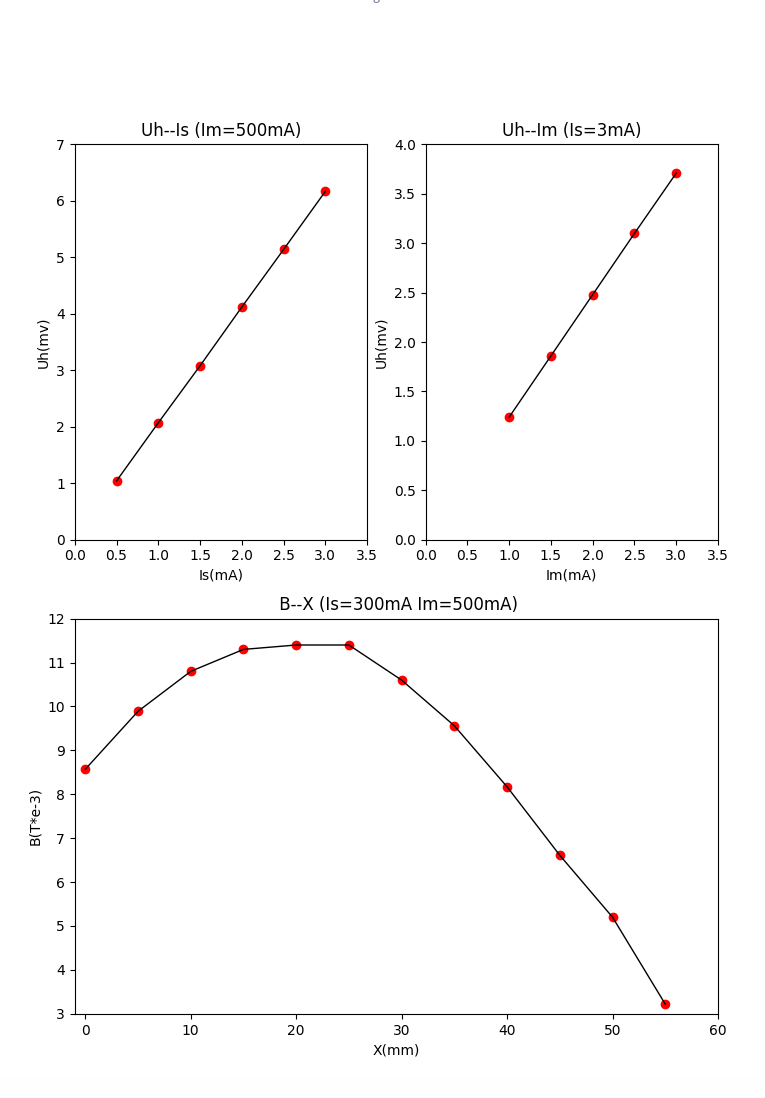第二个实验是单缝衍射光强分布的测定,一个图,需要将点光滑的连起来:

import numpy as np
import matplotlib.pyplot as plt
from scipy.interpolate import spline

plt.figure(figsize=(10,7))
x = np.arange(-16,15.5,0.5)
y = np.array(
[0.111  ,0.096, 0.101,  0.125,  0.166,  0.201,  0.246,  0.261,  0.266,  0.236,
0.201,  0.17,   0.175,  0.19,   0.276,  0.409,  0.572,  0.694,  0.787,  0.777,
0.72,   0.679,  0.788,  1.245,  3.535,  5.755,  6.945,  8.075,  10.635, 13.545,
16.025, 17.905, 18.705, 18.505, 17.155, 15.275, 12.625, 9.285,  7.075,  4.735,
2.935,  1.665,  1.025,  0.756,  0.749,  0.83,   0.832,  0.696,  0.507,  0.508,
0.428,  0.31,   0.252,  0.244,  0.261,  0.279,  0.28,   0.252,  0.191,  0.163,
0.129,  0.113,  0.114]);

xnew = np.linspace(x.min(),x.max(),1000)
power_smooth = spline(x,y,xnew) #将两点平滑的连接

plot1 = plt.plot(x, y, '*',label='original values')
plot2 = plt.plot(xnew, power_smooth, 'r',label='curve_fit values')
plt.xlabel('x/mm')
plt.ylabel('y/1e-7A')
plt.legend(loc=1)       #指定legend的位置
plt.hlines(0, -17, 16, linestyles = "dashed")   #画一条表示y=0的虚线
plt.title('Physical work')
plt.savefig('1.png')
plt.show()

结果如图: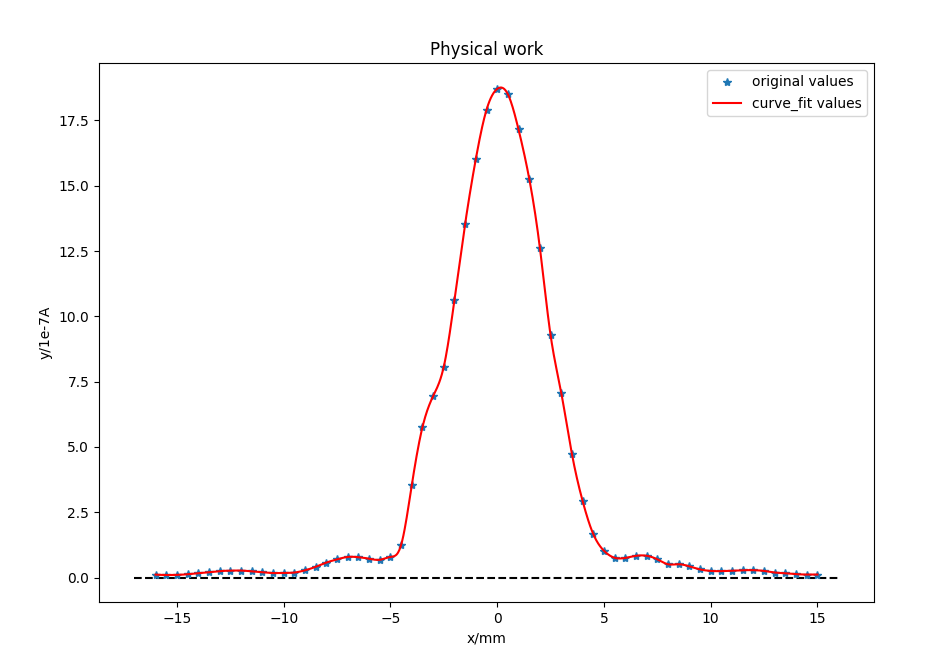第三个实验磁场的测量,需要四个图,而且要在图中显示中文:

import numpy as np
import matplotlib.pyplot as plt
from scipy.interpolate import spline
from matplotlib.font_manager import *
myfont = FontProperties(fname='/usr/share/fonts/truetype/wqy/wqy-microhei.ttc')  #指定字体配置(后面为你的系统中字体的位置)

plt.figure(figsize=(12,12))
plt.subplot(221)
x1 = np.arange(-50,60,10)
y1 = np.array([0.107, 0.118, 0.128, 0.136, 0.140, 0.142, 0.137,
0.131, 0.123, 0.112, 0.101])
y2 = np.array([0.106, 0.117, 0.128, 0.136, 0.142, 0.144, 0.142,
0.136, 0.128, 0.117, 0.106])
xnew = np.linspace(x1.min(),x1.max(),100)
power_smooth1 = spline(x1,y1,xnew)
power_smooth2 = spline(x1,y2,xnew)

plt.plot11 = plt.plot(x1, y1, 'ro')
plt.plot12 = plt.plot(xnew, power_smooth1)
plt.plot21 = plt.plot(x1, y2, 'ro')
plt.plot22 = plt.plot(xnew, power_smooth2)
plt.xlabel('x/mm')
plt.ylabel('y/mT')
plt.title('单个线圈轴线',fontproperties=myfont)   #指定字体
plt.xlim(-60,60)
plt.ylim(0.000,0.200)

plt.subplot(222)
x1 = np.arange(-50,60,10)
y1 = np.array([0.192, 0.197, 0.200, 0.201, 0.201, 0.201, 0.201,
0.201, 0.200, 0.197,0.192])
plt.plot(x1,y1,'-')
plt.plot(x1,y1,'ro')
plt.xlabel('x/mm')
plt.ylabel('y/mT')
plt.title("双线圈轴线",fontproperties=myfont)
plt.xlim(-60,60)
plt.ylim(0.000,0.210)

plt.subplot(223)
x1 = np.arange(-30,40,10)
y1 = np.array([0.201, 0.201, 0.201, 0.201, 0.201, 0.201, 0.200])
plt.plot(x1,y1,'-')
plt.plot(x1,y1,'ro')
plt.xlabel('x/mm')
plt.ylabel('y/mT')
plt.title("双线圈径线",fontproperties=myfont)
plt.xlim(-40,40)
plt.ylim(0.000,0.210)

plt.subplot(224)
x1 = np.arange(20,140,10)
y1 = np.array([0.194, 0.198, 0.202, 0.199, 0.200, 0.201, 0.201,
0.201, 0.199, 0.200, 0.201, 0.201])
plt.plot(x1,y1,'-')
plt.plot(x1,y1,'ro')
plt.xlabel('x/Hz')
plt.ylabel('y/mT')
plt.title("坐标原点处",fontproperties=myfont)
plt.xlim(0,140)
plt.ylim(0.000,0.210)
plt.show()


结果如图: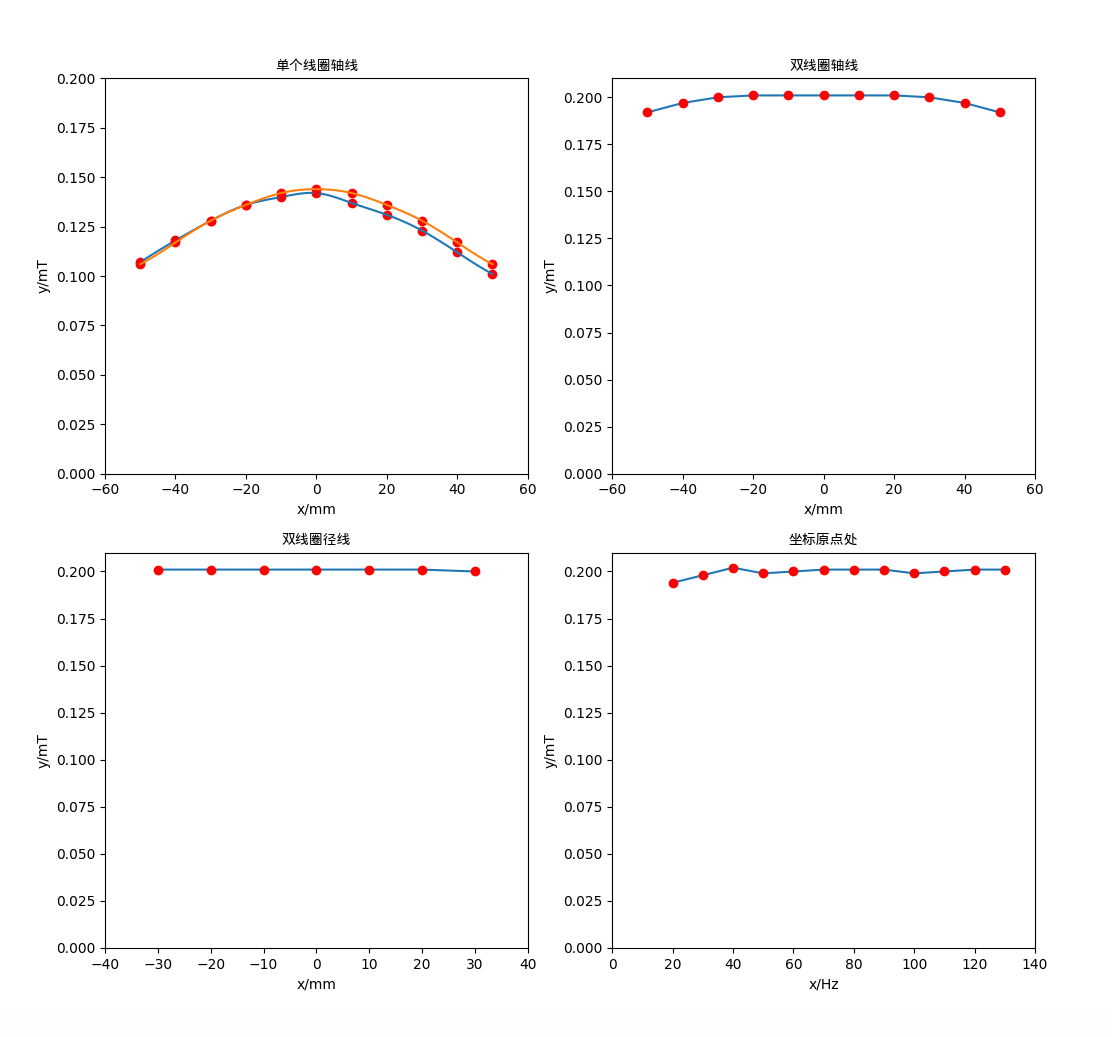展开全文• Python笔记—matplotlib雷达图 重点 实例代码： import numpy as np import matplotlib.pyplot as plt plt.rcParams['font....name = ['语文','数学','英语','物理','化学'] #标签 theta = np.linspace(0,2*n...
• 流线图可以被用来可视化矢量场的流态。如科学和自然学科中的磁场、万有引力和流体运动等均可以用流线图表示。矢量场通过为每个点指定一个线条和一个或多个箭头的...这时就需要使用matplotlib来画这样的流线图了。 .python
• 如果作为一个物理老师，也需要讲述力向量的方向和合成。如果需要现场可以动态在修改示意图时，你再采用预先绘制的方式，就不会适用，这时就可以采用matplotlib来动态编程，以便课堂上动态在修改，也可以分发给学生去...python
• 迈克尔孙干涉仪，是1881年美国物理学家迈克尔逊和莫雷合作，为研究“以太”漂移而设计制造出来的精密光学仪器。它是利用分振幅法产生双光束以实现干涉。通过调整该干涉仪，可以产生等厚干涉条纹 ，也可以产生等倾...python 计算机视觉
• 能够方便地绘图是MATLAB一大优势，但这方面现在并不领先Python。 以环境物理学原理课一次作业为例，除去数据处理部分，使用matplotlib.pyplot结合一点pandas，绘制出不输MATLAB的常用曲线图。python pyplot
• 我在Python 3.4.2中运行了示例代码，具有numpy版本1.9.1和matplotlib版本1.4.2，在具有4个物理CPU的Macbook Pro上(即，与“虚拟”CPU相反，Mac硬件架构也是提供一些用例)：import numpy as npimport matplotlib.mlab...
• 在自然界、物理学、生物学、化学和经济领域，随机漫步都有其实际用途。例如：漂浮在水滴上的花粉因不断受到水分子的挤压而在水面上移动。水滴中的分子运动是随机的，因此花粉在水面的运动路径犹如随机漫步。好了，接...
• 当我们要显示数据时，偶尔会遇到这样的问题，比如一条连续的曲线，由于物理条件限制，导致在某一个区域里不能取值，那么画曲线的时候，中间不能有值，也不能用连线连接起来。这时需要怎么处理呢？如果只是删除中间的...python
• 当我们想要使用坐标系来表示数据的关系时，往往需要给坐标系进行命名，比如物理学上的时间-距离曲线，就会把X轴命名为时间，Y轴命名为距离，这样画出来的曲线就是时间-距离曲线。当我们想表示收入-时间曲线时，可以...python
• 随机漫步在本节中，我们将使用Python生成随机漫步数据，再使用matplotlib以引入瞩目的方式将这些数据呈现出来。随机漫步是这样行走得到的路径：每次行走都完全随机的，没有明确的方向，结果是由一系列随机决策决定的...python随机漫步
• 我安装的是python2.7——64位，在这里建议大家安装64位，32位存在一个内存限制的问题，如果处理数据量较少则没什么问题，一旦处理数据量较大，则会出现物理内存足够，但为什么还是报内存溢出的错误，这就是版本的...
• 信号是随时间和空间变化的某种物理量，它一般是时间变量t的函数。信号随时间变量t变化的函数曲线成为信号的波形。 本部分主要内容包括两块: 信号的时域变换 信号的基本运算 下面我将用Python+matplotlib的方式绘制...python 可视化 信号与系统 时域分析
• 由于这样的原因,我经常避免使用Basemap,并使用OGR读取shapefile,并将其自己转换为Matplotlib艺术家.哪些是更多的工作,但也有更多的灵活性.底图具有一些非常整洁的功能,例如将输入数据的坐标转换为“工作投影”.如果...
• 地震折射数据集的使用对于近地表地球物理勘探至关重要，并且经常用于描述基岩的深度。 该项目将利用Obspy python框架读取SEG2折射数据集，并使用matplotlib.pyplot.ginput函数绘制交互式流数据集。 目录 安装 ...
• ”——物理学家惠勒 分形理论是在上世纪70年代由芒德布罗几乎集一己之力创立的，但其严格的数学基础之一——芒德布罗集，却是70年代末芒德布罗及布鲁克斯、马蒂尔斯基以及道阿迪、哈伯德、沙斯顿等人几乎同时分别...python
• SciPy: 基于Numpy，提供方法(函数库)直接计算结果，封装了一些高阶抽象和物理模型。比方说做个傅立叶变换，这是纯数学的，用Numpy；做个滤波器，这属于信号处理模型了，在Scipy里找。 Pandas: 提供了一套名为...
• 随机漫步（Random walk)，也称随机...这个概念在1905年由卡尔·皮尔逊首次提出，目前已在许多领域中得到应用，如生态学、经济学、心理学、计算科学，物理学、化学和生物学等。 随机漫步有很多实际用途。比如，分子...Python 数据分析 数据可视化
• SciPy：基于Numpy，提供方法(函数库)直接计算结果，封装了一些高阶抽象和物理模型。比方说做个傅立叶变换，这是纯数学的，用Numpy；做个滤波器，这属于信号处理模型了，在Scipy里找。 Pandas：提供了一套名为...
• SciPy: 基于Numpy，提供方法(函数库)直接计算结果，封装了一些高阶抽象和物理模型。比方说做个傅立叶变换，这是纯数学的，用Numpy；做个滤波器，这属于信号处理模型了，在Scipy里找。Pandas: 提供了一套名为...numpy 合并
• 1.帕累托分布帕累托分布是一种幂律概率分布，以意大利土木工程师、经济学家和社会学家维尔弗雷多·帕累托的名字命名，用来描述社会、科学、地球物理、精算和其他各种可观察到的现象。帕累托分配有时也被称为帕累托...
• 机械能是动能与势能的总和，这里的势能分为重力势能...机械能是表示物体运动状态与高度的物理量。物体的动能和势能之间是可以转化的。在只有动能和势能相互转化的过程中，机械能的总量保持不变，即机械能是守恒的。 ...opencv读取图片 opencv轮廓匹配
• 科学计算学习·总序 终于终于来到了这一步，Python的一大重要应用领域在于科学计算，而正是博主所需要具备的能力。学习教材强推张若愚的《Python科学计算(第二版)》。...刻画物理演化过程的方程，均是微分方程，...科学计算 python
• 在自然界、物理学、生物学、化学和经济领域，随机漫步都有其实际用途。例如，漂浮在水滴上的花粉因不断受到水分子的挤压而在水面上移动。水滴中的分子运动是随机的，因此花粉在水面上的运动路径犹如随机漫步。python 列表
• matplotlib 3.1.3 numpy的1.18.1 枕头7.1.2 摘要迄今为止，为光学计算成像（CI）提出的大多数神经网络都采用监督训练策略，因此需要大量训练来优化其权重和偏差。 在许多实际应用中，在许多小时的数据采集中，...Python